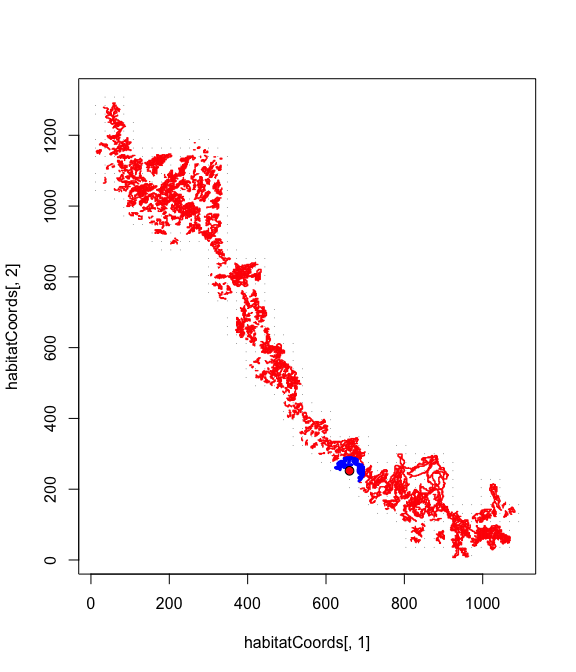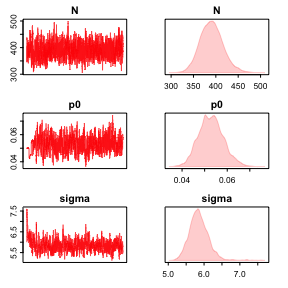# Wolverine Example

#### 2022-11-30

This vignette demonstrates the wolverine spatial capture-recapture model, as in “Efficient MCMC for Spatial Capture-Recapture Models” (Turek et al, submitted). Specifically, we implement the final version of the wolverine model and MCMC using nimble (de Valpine et al. 2017; NIMBLE Development Team 2020). Details of the functions and procedure are provided therein.

library(nimble)
library(basicMCMCplots)
library(coda)

We compiled all functions in the nimbleSCR package version 0.1.0 (Bischof et al. 2020).

library(nimbleSCR)

### Define Model Structure

Here, we define the nimble model structure.

code <- nimbleCode({
## priors
psi ~ dunif(0, 1)
sigma ~ dunif(0, 50)
p0 ~ dunif(0, 1)
## loop over individuals
for(i in 1:n.individuals) {
## AC coordinates
sxy[i,1] ~ dunif(0, x.max)
sxy[i,2] ~ dunif(0, y.max)
## habitat constraint
ones[i] ~ dHabitatMask( s = sxy[i,1:2],
xmin = lowerCoords,
xmax = upperCoords,
ymin = lowerCoords,
ymax = upperCoords,
habitat = habitat.mx[1:y.max,1:x.max])
z[i] ~ dbern(psi)
## likelihood
y[i, 1:nMaxDetectors] ~ dbinomLocal_normal( detNums = nbDetections[i],
detIndices = yDets[i,1:nMaxDetectors],
size = trials[1:n.detectors],
p0 = p0,
s = sxy[i,1:2],
sigma = sigma,
trapCoords = detector.xy[1:n.detectors,1:2],
localTrapsIndices = detectorIndex[1:n.cells,1:maxNBDets],
localTrapsNum = nDetectors[1:n.cells],
resizeFactor = ResizeFactor,
habitatGrid = habitatIDDet[1:y.maxDet,1:x.maxDet],
indicator = z[i])
}
## derived quantity: total population size
N <- sum(z[1:n.individuals])
})

We load the wolverine example data available from the Dryad Digital Repository (Milleret et al. 2018). See Milleret et al. (2019) for a complete description of the data.

We also create objects code, constants, data, and inits for later use in the function nimbleModel.

load("WolverineData.RData")
data <- list(y = my.jags.input$y, z = my.jags.input$z,
detector.xy = my.jags.input$detector.xy, habitat.mx = my.jags.input$habitat.mx,
ones = my.jags.input$OK, lowerCoords = c(0,0), upperCoords = c( dim(my.jags.input$habitat.mx),
dim(my.jags.input$habitat.mx)), trials = rep(1, dim(my.jags.input$detector.xy)))
constants <- list(n.individuals = my.jags.input$n.individuals, n.detectors = dim(my.jags.input$detector.xy),
y.max = dim(my.jags.input$habitat.mx), x.max = dim(my.jags.input$habitat.mx))
inits <- list(sxy = inits.1$sxy, z = inits.1$z,
p0 = 0.05,
psi = 0.5,
sigma = 6)

## Use of custom distribution to increase MCMC efficiency

The dbinomLocal_normal() distribution incorporates three features to increase computational efficiency.

### 1. Local detector evaluation

This step restricts calculations of the detection probabilities (here using a halfnormal function) to detectors (traps) within a radius where detections are realistically possible (Milleret et al. 2019). We use the function getLocalObjects to identify the set of detectors that are within a certain distance $$d_{max}$$ of each habitat cell center (blue points in the plot below). These reduced sets of detectors are stored in the localIndices matrix and are later used in the local evaluation of the detection model to speed up calculations. The value of $$d_{max}$$ should be as small as possible in order to reduce computation but always large enough so that for any particular individual, the set of local traps associated with the coordinates of the activity center s include all detectors at which that individual was detected. The $$d_{max}$$ value will therefore affect the number of columns in localIndices Here, we use $$d_{max}=38$$. with the coordinates of the activity center s … We also aggregated the habitat matrix to obtain larger habitat cells (lower resolution) and obtain objects with smaller dimensions. This reduces the number of habitat cells for which we have to identify the set of detectors that are within $$d_{max}$$ of the cell center. The goal is to create the object localIndices of the smallest dimension possible, that balances the cost of looking up relevant grid cells and reducing calculations for each grid cell.

Here, we resize the habitat matrix by a factor of 24, which corresponds to the resizeFactor argument. This means that 24x24 cells are aggregated into a single cell. The resizeFactor value will affect how many rows localIndices will be composed of.

set.seed(2)

DetectorIndex <- getLocalObjects(habitatMask = data$habitat.mx, coords = data$detector.xy,
dmax = 38,
resizeFactor = 24)constants$y.maxDet <- dim(DetectorIndex$habitatGrid)
constants$x.maxDet <- dim(DetectorIndex$habitatGrid)
constants$ResizeFactor <- DetectorIndex$resizeFactor
constants$n.cells <- dim(DetectorIndex$localIndices)
constants$maxNBDets <- DetectorIndex$numLocalIndicesMax
data$detectorIndex <- DetectorIndex$localIndices
data$nDetectors <- DetectorIndex$numLocalIndices
data$habitatIDDet <- DetectorIndex$habitatGrid

### 2. Sparse representation of the observation matrix

We re-express y as a sparse representation of the detection matrix to reduce its size. In this representation, we turn the detection matrix y into three objects:

• detIndices: where each row (corresponding to one individual) contains the identification numbers of detectors at which that individual was detected.
• y: a second matrix of identical dimension, containing the number of detections of a given individual at each detector. This second matrix is necessary for modelling non-binary detections (e.g. $$binomial$$ observation models)
• detNums: a vector containing the number of detectors at which each individual was detected.
ySparse <- getSparseY(x = my.jags.input$y) data$y <- ySparse$y[,,1] data$yDets <- ySparse$detIndices[,,1] data$nbDetections <- ySparse$detNums[,1] constants$nMaxDetectors <- ySparse$maxDetNums ### 3. Skip unnecessary calculations The function dbinomLocal_normal() takes the logical argument indicator that specifies whether the individual i is available ($$z_i$$ = 1) for detection or not ($$z_i$$ = 0). When $$z_i$$ = 0, calculations of $$p_{ij}$$ are not performed and therefore increases MCMC efficiency. ## Create NIMBLE Model Now, we can create the nimble model object, using the model structure defined in code, and the constants, data, and initial values. Rmodel <- nimbleModel(code, constants, data, inits) #### Configure and Build MCMC We configure an MCMC algorithm to the Rmodel model object. We assign MCMC monitors to $$N$$, $$\sigma$$, and $$p_0$$. #### Block sampling to increase MCMC efficiency We also remove the univariate Metropolis-Hastings samplers that were assigned by default to each dimension of the sxy variables (ACs). Instead, we add joint Metropolis-Hastings samplers (RW_block) samplers to each $$x$$ and $$y$$ coordinate pair sxy[i, 1:2]. conf <- configureMCMC(Rmodel, monitors = c("N", "sigma", "p0"), print = FALSE) conf$removeSamplers("sxy")
ACnodes <- paste0("sxy[", 1:constants$n.individuals, ", 1:2]") for(node in ACnodes) { conf$addSampler(target = node,
type = "RW_block",
silent = TRUE)
}
Rmcmc <- buildMCMC(conf)

#### Compile and Run MCMC

Finally, we compile both the model and MCMC objects and execute the compiled MCMC for 10 000 iterations.

Cmodel <- compileNimble(Rmodel)
Cmcmc <- compileNimble(Rmcmc, project = Rmodel)
MCMC_runtime <- system.time(
samples <- runMCMC(Cmcmc, niter = 10000)
)

## Results

First, we can extract the MCMC runtime (5.2 minutes in this case):

round(MCMC_runtime / 60, 1)
## elapsed
##     5.2

Next, we can check the posterior effective sample size (ESS) resulting from our 10 000 posterior samples for the three parameters we tracked ($$N$$, $$\sigma$$, and $$p_0$$):

round(effectiveSize(samples),2) 
##      N     p0  sigma
## 463.24 290.02 179.18

We can also calculate the MCMC efficiency for each parameter; this corresponds to the rate of generating effectively independent posterior samples, per second of MCMC runtime:

round(effectiveSize(samples)/MCMC_runtime,2)  
##     N    p0 sigma
##  1.48  0.93  0.57

Summary of posterior distributions for each parameter:

round(samplesSummary(samples), 2)
##         Mean Median St.Dev. 95%CI_low 95%CI_upp
## N     390.54 390.00   25.34    343.00    444.00
## p0      0.05   0.05    0.01      0.04      0.06
## sigma   5.86   5.84    0.27      5.38      6.40

Examine traceplots and posterior distributions:

chainsPlot(samples)